# Methods and formulas for the tolerance intervals in Tolerance Intervals (Nonnormal Distribution)

Select the method or formula of your choice.

## Tolerance interval methods

Minitab calculates both parametric and nonparametric tolerance intervals. The calculations for the nonparametric tolerance intervals assume only that the parent distribution is continuous. The calculations for the parametric tolerance intervals assume that the parent distribution of the sample is one of these distributions:
• Lognormal
• Gamma
• Exponential
• Smallest extreme value
• Weibull
• Largest extreme value
• Logistic
• Loglogistic

### General Definitions

Let X 1, X 2, ..., X n be the ordered statistics based on random sample of size n from some continuous distribution.

Let the distribution function be F(x;θ) for Ω in some parameter space with dimension greater than or equal to 1.

Let L < U be two statistics based on the sample such that for any given values α and P, with 0 < α < 1 and 0 < P < 1, the following holds for every θ in Ω: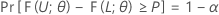Then, the interval [L, U] is a two-sided tolerance interval with content = P x 100% and confidence level = 100(1 – α)%. Such an interval can be called a two-sided (1 – α, P) tolerance interval. For example, if α = 0.10 and P = 0.85, then the resulting interval is called a two-sided (90% , 0.85) tolerance interval.

If L = –∞ and U < +∞, then the interval (-∞, U] is called a one-sided (1 – α, P) upper tolerance bound. If L > -∞ and U = +∞, then the interval [L, +∞) is called a one-sided (1 – α, P) lower tolerance bound.

Tolerance intervals possess the following interesting and useful properties:
• A one-sided (1 – α, P) lower tolerance bound is also a one-sided (P, 1 – α) upper tolerance bound.
• A one-sided (1 – α )100% lower confidence bound of the (1 – P)th percentile of the distribution of the data is also a one-sided (1 – α, P) lower tolerance bound. Similarly, a one-sided (1 – α )100% upper confidence bound of the P th percentile of the distribution of the data is also a one-sided (1 – α , P) upper tolerance bound.
• If L and U are one-sided (1 – α/2 , (1 + P )/2) lower and upper tolerance bounds, then [ L, U ] is an approximate two-sided (1 – α, P ) tolerance interval. This method may be used in cases where two-sided tolerance intervals cannot be directly obtained. The resulting two-sided tolerance intervals are generally conservative. See Guenther1 and Hahn and Meeker2.
1. Guenther, W. C. (1972). Tolerance intervals for univariate distributions. Naval Research Logistics, 19: 309–333.
2. Hahn G. J. and Meeker W. Q. (1991). Statistical Intervals: A Guide for Practitioners John Wiley & Sons, New York.

## Exact nonparametric tolerance intervals for continuous distributions

Minitab calculates exact (1 – α, P) nonparametric tolerance intervals, where 1 – α is the confidence level and P is the coverage (the target minimum percentage of population in the interval). The nonparametric method for tolerance intervals is a distribution free method. That is, the nonparametric tolerance interval does not depend on the parent population of your sample. Minitab uses an exact method for both one-sided and two-sided intervals.

Let X 1, X 2 , ... , X n be the ordered statistics based on a random sample from some continuously distributed population F(x;θ). Then, based on the findings of Wilks1, 2 and Robbins3, it can be shown that: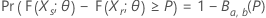where B denotes the cumulative distribution function of the beta distribution with parameters a = r and b = ns + 1. Thus ( Xr , Xs ) is a distribution-free tolerance interval because the coverage of the interval has a beta distribution with known parameter values, which are independent of the distribution of the parent population, F(x;θ).

### One-sided intervals

Let k be the largest integer that satisfies the following: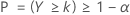where Y is a binomial random variable with parameters n and 1 – P. It can be shown (see Krishnamoorthy and Mathew4) that a one-sided (1 – α, P) lower tolerance bound is given by Xk . Similarly, a one-sided (1 – α, P) upper tolerance bound is given by X n - k +1. In both cases, the actual or effective coverage is given by P(Y > k).

### Two-sided intervals

Let k be the smallest integer that satisfies the following: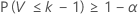where V is a binomial random variable with parameters n and P. Thus,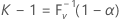where F V -1(x) is the inverse cumulative distribution function of V. It can be shown (see Krishnamoorthy and Mathew4) that a two-sided (1 – α, P) tolerance interval may be given as ( Xr , Xs ). Minitab chooses s = n - r + 1 so that r = ( nk + 1) / 2. Both r and s are rounded down to the nearest integer. The actual or effective coverage is given by P(V < k – 1).

### Notation

TermDescription
1 – αthe confidence level of the tolerance interval
P the coverage of the tolerance interval (the target minimum percentage of population in the interval)
n the number of observations in the sample
1. Wilks, S. S. (1941). Sample size for tolerance limits on a normal distribution. The Annals of Mathematical Statistics, 12, 91–96.
2. Wilks, S. S. (1941). Statistical prediction with special reference to the problem of tolerance limits. The Annals of Mathematical Statistics, 13, 400–409.
3. Robbins, H. (1944). On distribution-free tolerance limits in random sampling. The Annals of Mathematical Statistics, 15, 214–216.
4. Krishnamoorthy, K. and Mathew, T. (2009). Statistical Tolerance Regions: Theory, Applications, and Computation. Wiley, Hoboken, NJ.

## Lognormal distribution

The tolerance interval for the lognormal distribution uses the same equations as tolerance intervals for the normal distribution. The calculations follow this process:
1. Minitab takes the natural logarithm of the data.
2. Minitab calculates a tolerance interval for the transformed data using the tolerance interval procedure for the normal distribution.
3. Minitab exponentiates the limits of the tolerance interval obtained in the previous step to transform the interval to the scale of the original data.
For formulas that apply to the normal distribution, go to Methods and formulas for Tolerance Intervals (Normal Distribution) and click "Exact tolerance intervals for normal distributions.".

## Approximate tolerance intervals for gamma distributions

The tolerance interval for the gamma distribution uses an approximation to the normal distribution. Krishnamoorthy, et al. conduct simulation studies that show that the approximation provides accurate results. The calculations follow this process:

1. Minitab takes the cubic root of the data.
2. Minitab calculates a tolerance interval for the transformed data using the tolerance interval procedure for the normal distribution.
3. Minitab exponentiates the limits of the tolerance interval obtained in the previous step to transform the interval to the scale of the original data.
For formulas that apply to the normal distribution, go to Methods and formulas for Tolerance Intervals (Normal Distribution) and click "Exact tolerance intervals for normal distributions."
1. Krishnamoorthy K., Mathew T and Mukherjee S (2008). Normal based methods for a Gamma distribution: prediction and tolerance intervals and stress-strength reliability. Technometrics, 50, 69—78.

## Exponential distribution

Minitab calculates exact (1 – α, P) tolerance intervals, where 1 – α is the confidence level and P is the coverage (the target minimum percentage of population in the interval). The formulas differ between the calculation of one-sided tolerance limits and two-sided tolerance intervals.

### One-sided exponential tolerance limits

This formula gives the lower limit:

This formula gives the upper limit:

### Two-sided exponential confidence intervals

Minitab uses Newton's method to solve the following system of equations. For more detail, see Fernandez1.

This formula gives the two-sided interval:

Where,

and the value of k 1 depends on the solution to this system of equations:

where,

### Notation

TermDescription
n the sample size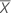the sample mean
P the target minimum percentage of the population in the interval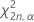the α th percentile of the chi-square distribution with 2n degrees of freedom
α 1 − confidence level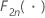the cumulative distribution function of the chi-square distribution with 2n degrees of freedom
1. Fernandez, Arturo J. (2010). Two-sided tolerance intervals in the exponential case: Corrigenda and generalizations. Computational Statistics and Data Analysis, 54, 151—162.

## Smallest extreme value distribution

Minitab calculates exact (1 – α, P) tolerance intervals based on Lawless1, where 1 – α is the confidence level and P is the coverage (the target minimum percentage of population in the interval).

### Exact one-sided smallest extreme value tolerance limits

This formula gives the lower limit:

where

and x is the unique root of this function:

with

where

and Cz is a normalizing constant:
Also, the function IGn [x] is the incomplete gamma function:
This formula gives the upper limit:

The value of k 2 comes from replacing α with 1 − α and P with 1 − P in the formulas for computing k 1.

### Approximate two-sided smallest extreme value tolerance intervals

To calculate the approximate two-sided interval, replace α by α/2 and P by (P + 1)/2 in the formulas for computing the one-sided tolerance limits.

### Notation

TermDescription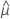the maximum likelihood estimate of the location parameter of the extreme value distribution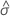the maximum likelihood estimate of the scale parameter of the extreme value distribution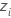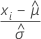, the centered observations based on the MLE estimates of the location and scale parameters of the smallest extreme value distribution
t the α th percentile of the non-central t-distribution with n − 1 degrees of freedom and noncentrality parameter δP
1 - α the confidence level of the tolerance interval
P the coverage of the tolerance interval (the target minimum percentage of population in the interval)
n the number of observations in the sample
1. Lawless, J. F. (1975). Construction of tolerance bounds for the extreme-value and the Weibull distribution. Technometrics, 17, 255—261.

## Weibull distribution

The tolerance interval for the Weibull distribution uses the same equations as tolerance intervals for the smallest extreme value distribution. The calculations follow this process:
1. Minitab takes the natural logarithm of the data.
2. Minitab calculates a tolerance interval for the transformed data using the tolerance interval procedure for the smallest extreme value distribution.
3. Minitab exponentiates the limits of the tolerance interval obtained in the previous step to transform the interval to the scale of the original data.
For formulas that apply to the smallest extreme value distribution, go to the section on the smallest extreme value distribution.

## Largest extreme value distribution

The tolerance interval for the largest extreme value distribution uses the same equations as tolerance intervals for the smallest extreme value distribution. The calculations follow this process:
1. Minitab multiplies the data by −1.
2. Minitab calculates a tolerance for the transformed data using the tolerance interval procedure for the smallest extreme value distribution.
3. Minitab exponentiates the limits of the tolerance interval obtained in the previous step to transform the interval to the scale of the original data.

For formulas that apply to the smallest extreme value distribution, go to the section on the smallest extreme value distribution.

## Logistic distribution

Minitab calculates approximate (1 – α, P) tolerance intervals based on Bain and Engelhardt1, where 1 – α is the confidence level and P is the coverage (the target minimum percentage of population in the interval). The formulas differ between the calculation of one-sided tolerance limits and two-sided tolerance intervals.

### One-sided logistic tolerance limits

This formula gives the lower limit:
This formula gives the upper limit:
This formula gives the tolerance factor, k, in these formulas for the limits:

where

### Two-sided logistic tolerance intervals

To calculate the approximate two-sided interval, replace α by α/2 and P by (P + 1)/2 in the formulas for computing the one-sided tolerance limits.

### Notation

TermDescription
nthe number of observations in the sample
z1−αthe 1 - α percentile of the standard normal distribution
q1–Pthe 1 – P percentile of the standard logistic distribution
C11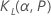C22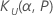C12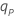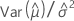the maximum likelihood estimate of the logistic location parameter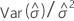the maximum likelihood estimate of the logistic scale parameter
1. Bain, L. and Englehardt, M. (1991). Statistical analysis of reliability and life testing models: Theory and Methods. Second edition, Marcel Dekker, Inc.

## Loglogistic distribution

The tolerance interval for the loglogistic distirbution uses the same equations as tolerance intervals for the logistic distribution. The calculations follow this process:
1. Minitab takes the natural logarithm of the data.
2. Minitab calculates a tolerance interval for the transformed data using the tolerance interval procedure for the logistic distribution.
3. Minitab exponentiates the limits of the tolerance interval obtained in the previous step to transform the interval to the scale of the original data.

For formulas that apply to the logistic distribution, go to the section on the logistic distribution.

## Anderson-Darling test

Minitab uses Anderson-Darling statistics to perform the goodness-of-fit test.

Let Z = F(X), where F(X) is the cumulative distribution function. Suppose that a sample X1, .., Xn gives values Z(i) = F(Xi), i=1,.., n. Rearrange Z(i) in ascending order, Z(1) < Z(2) <...<Z(n). Then the Anderson-Darling statistic (A2) is calculated as follows:

• A2 = –n - (1/n) Σi[(2i – 1) log Z(i) + (2n + 1 – 2i) log (1 – Z(i))]

The modified Anderson-Darling goodness-of-fit test statistic is calculated for each distribution. The p-values are based on the table given by D'Agostino and Stephens.1 If no exact p-value is found in the table, Minitab calculates the p-value based on interpolation using the range of the p-value.

1 R.B. D'Agostino and M.A. Stephens (1986). Goodness-of-Fit Techniques. Marcel Dekker.
By using this site you agree to the use of cookies for analytics and personalized content.  Read our policy